Geometry

# Tangent and Secant Lines: Level 2 Challenges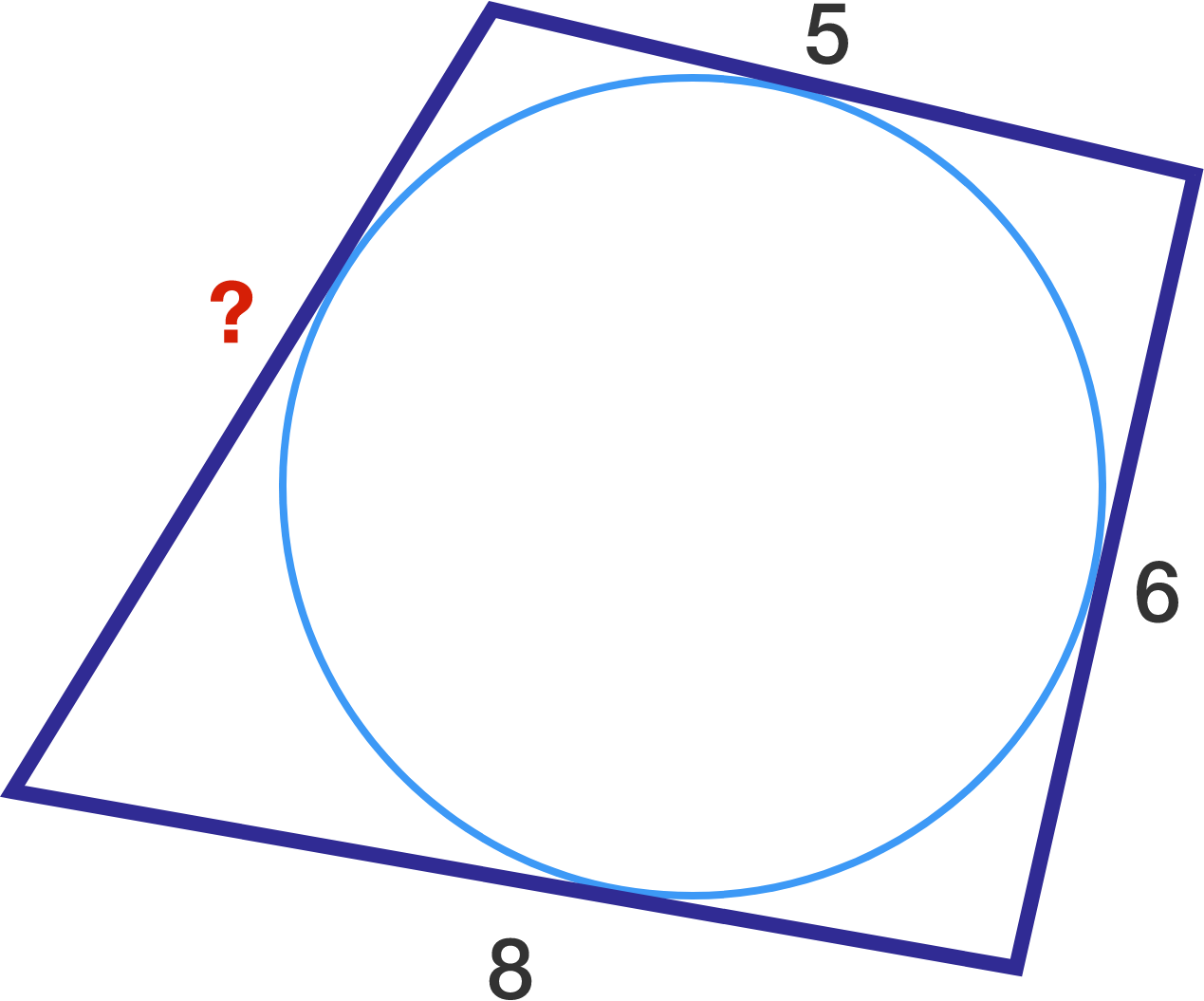Given the quadrilateral and inscribed circle, what is the missing side length?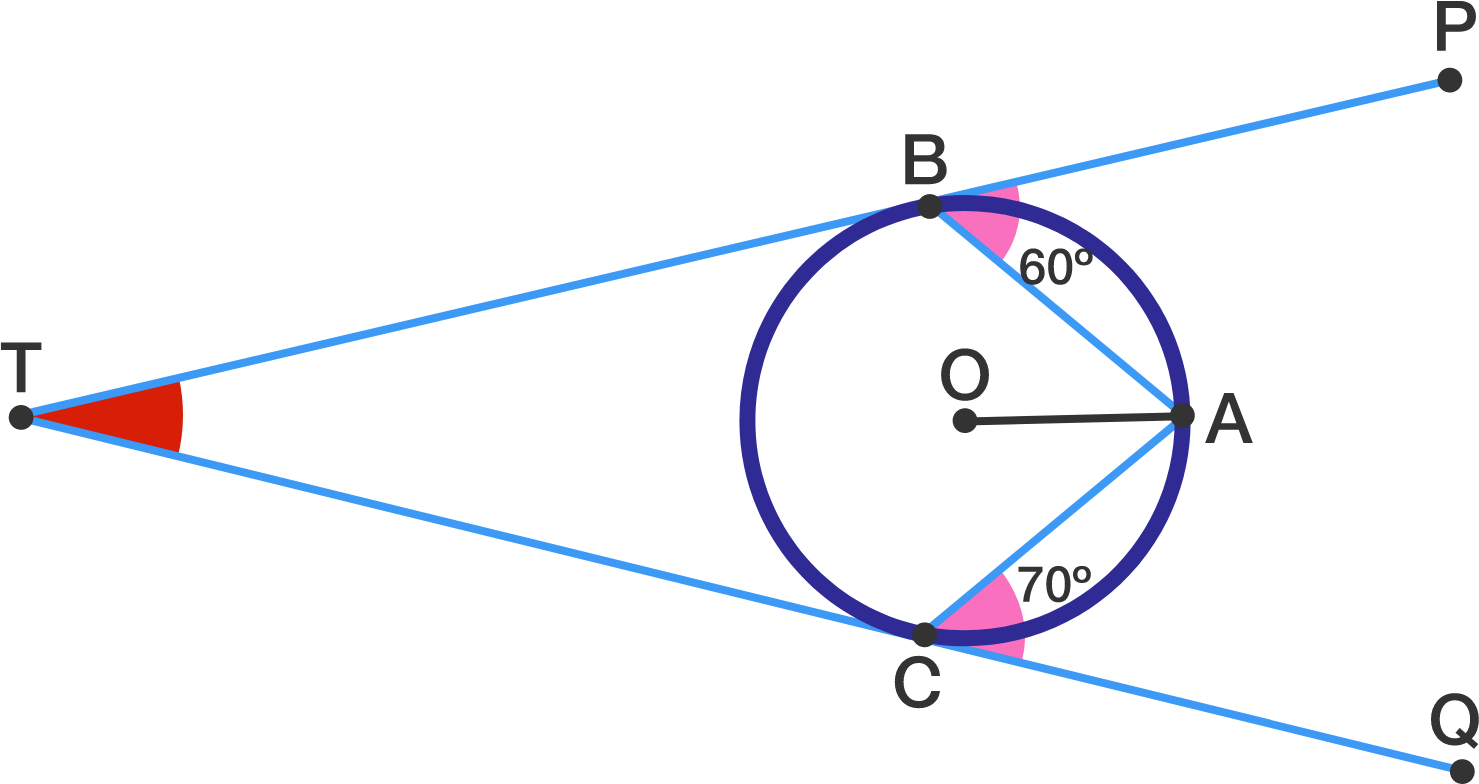In the figure given above $$\overline{TP}$$ and $$\overline{TQ}$$ are tangent to the circle with center $$O$$ at $$B$$ and $$C,$$ respectively.

If $$\angle PBA=60^\circ$$and $$\angle ACQ=70^\circ$$, what is $$\angle BTC$$ in degrees?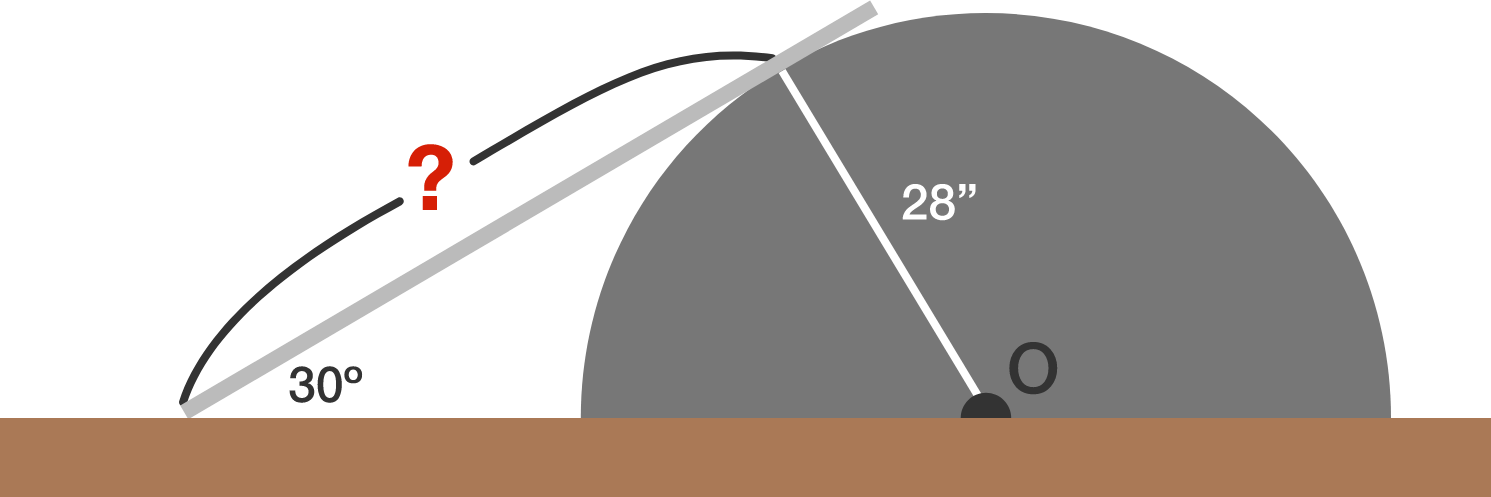There is a semi-circular object on a road and a strong metal plate is put on it, as shown in the above diagram, so that cars can better pass over it. If the radius of the object is $$28$$ inches and the angle between the plate and the road is $$30^\circ,$$ what is the distance (in inches) between the point the plate meets the road and the point the plate touches the semi-circular object?

A point $$P$$ is given outside of a circle $$\Gamma$$. A tangent from $$P$$ touches $$\Gamma$$ at $$T$$ with $$PT=45$$. A line from $$P$$ cuts $$\Gamma$$ at the 2 points $$A, B$$. If $$PA=25$$, what is the length of $$PB$$?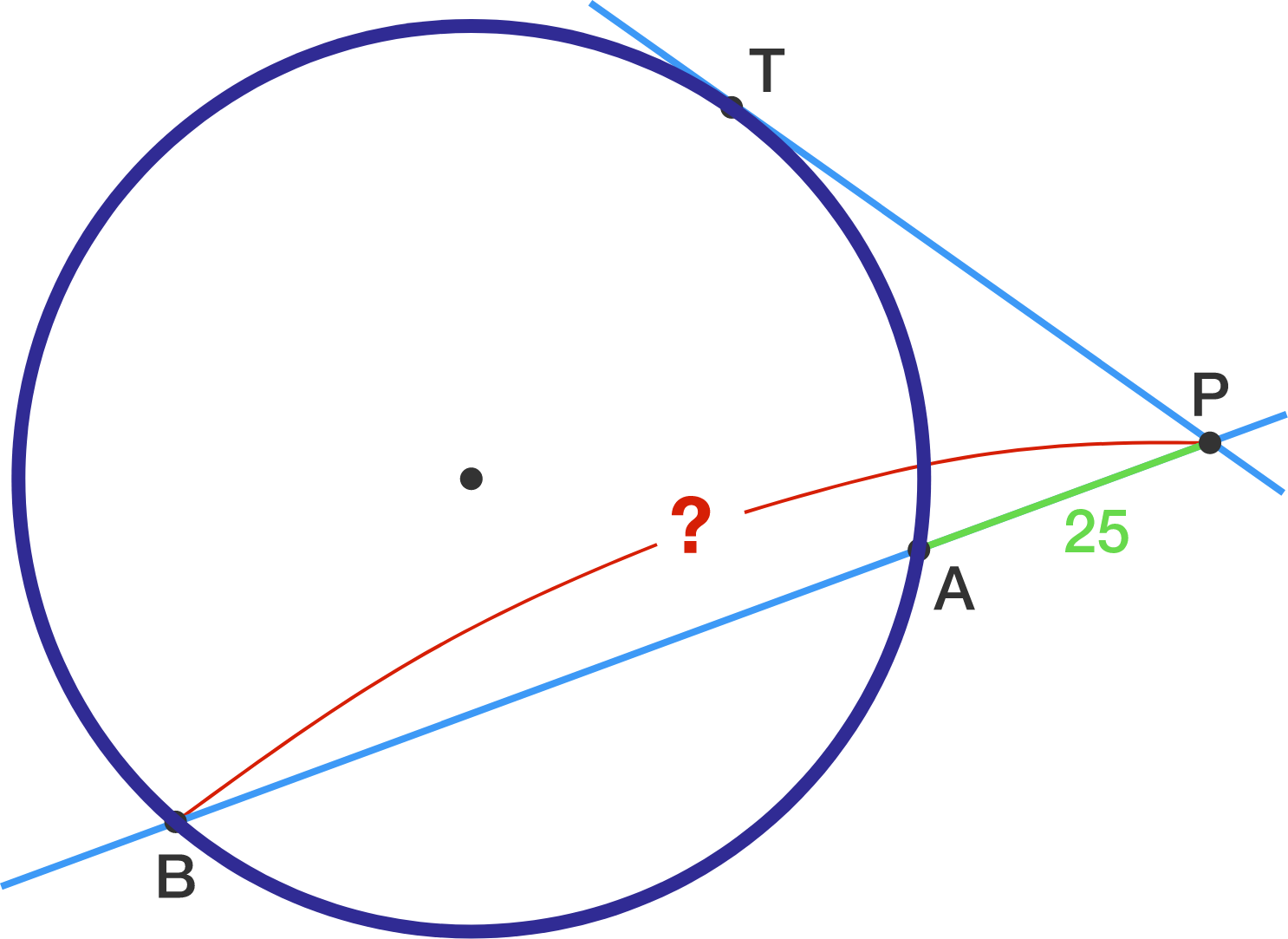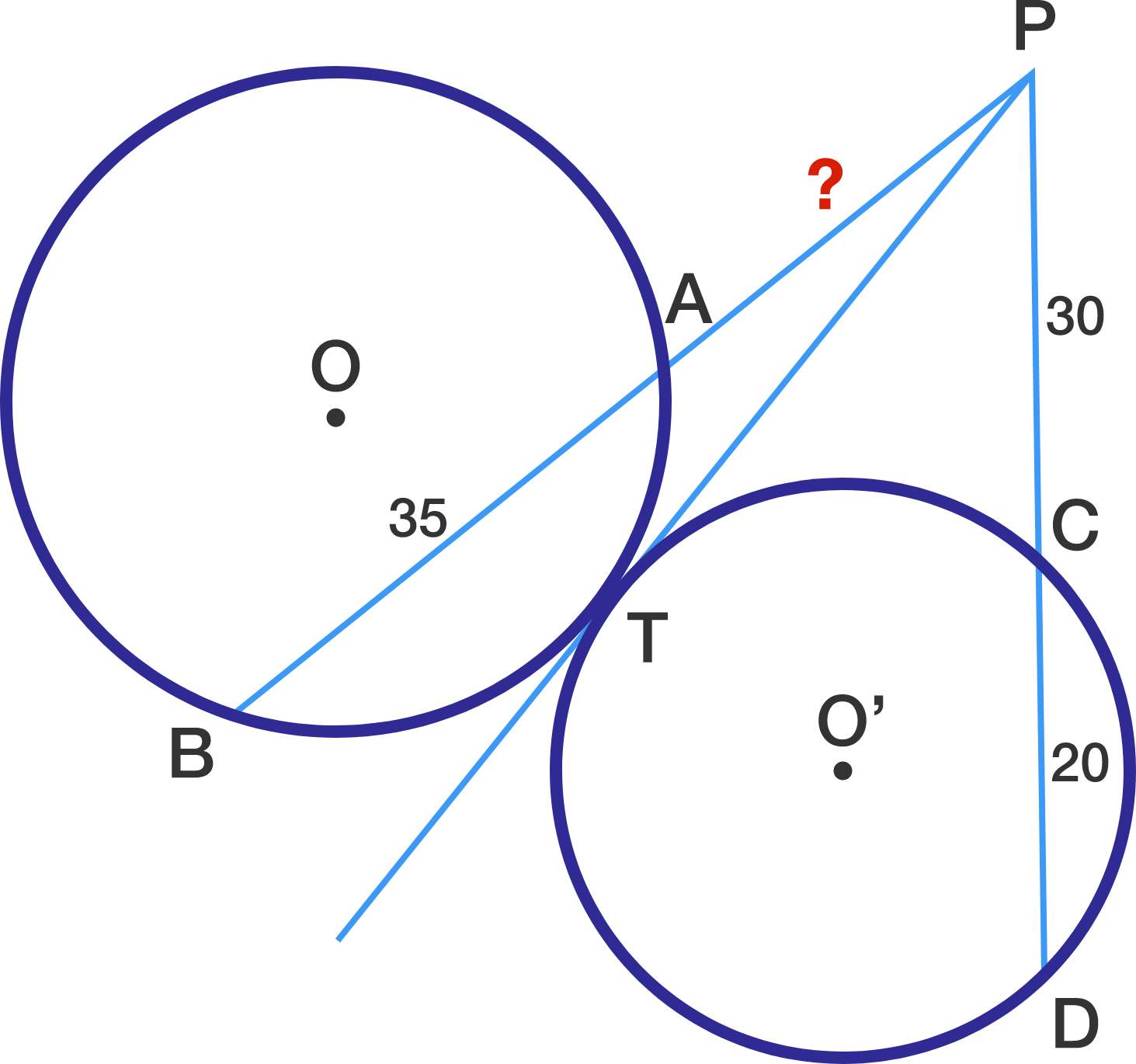In the above diagram, line segment $$\overline{PT}$$ is tangent to both circle $$O$$ and circle $$O'.$$ Given the following three lengths: $\lvert\overline{AB}\rvert = 35, \lvert\overline{PC}\rvert = 30, \lvert\overline{CD}\rvert = 20,$ what is $$\lvert\overline{PA}\rvert?$$

×

Problem Loading...

Note Loading...

Set Loading...